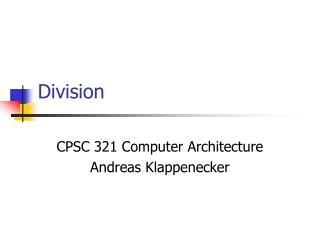# Division - PowerPoint PPT PresentationDownload PresentationDivision

Presentation Description
Download Presentation## Division

- - - - - - - - - - - - - - - - - - - - - - - - - - - E N D - - - - - - - - - - - - - - - - - - - - - - - - - - -
##### Presentation Transcript

1. Division CPSC 321 Computer Architecture Andreas Klappenecker

2. Division 1001 Quotient Divisor 1000 1001010 Dividend–1000 10 101 1010–1000 10 Remainder

3. Binary division • Dividend = Quotient x Divisor + Remainder • See how big a number can be subtracted • Binary division is simpler Create a quotient bit (0 or 1) in each step

4. rem = rem - div if rem < 0 then // divisor too big rem = rem + div quo <<= 1 LSB(quo) = 0 else // can divide quo <<= 1 LSB(quo) = 1 fi div >>= 1 repeat unless done

5. Division hardware (Version 1)

6. Binary division

7. Observations • Half of the bits in divisor always 0 • Half of 64 bit adder wasted • Half of divisor is wasted • Instead of shifting divisor to the right, shift remainder to the left • Shift left first to save one iteration

8. Division hardware (Version 2) ALU and divisor registers are reduced, remainder shifted left

9. Start: Place Dividend in Remainder 1. Shift the Remainder register left1 bit. 2.Subtract the Divisor register from the left half of the Remainder register, & place the result in the left half of theRemainder register. 3b. Restore the original value by adding the Divisor register to the left half of the Remainder register, &place the sum in the left half of the Remainder register. Also shift the Quotient register to the left, setting the new least significant bit to 0. 3a. Shift the Quotient register to the left setting the new rightmost bit to 1. nth repetition? Done Remainder > 0 Test Remainder Remainder < 0 No: < n repetitions Yes: n repetitions (n = 4 here)

10. Observations on Divide Version 2 • Eliminate Quotient register by combining with Remainder as shifted left • Start by shifting the Remainder left as before. • Thereafter loop contains only two steps because the shifting of the Remainder register shifts both the remainder in the left half and the quotient in the right half • The consequence of combining the two registers together and the new order of the operations in the loop is that the remainder will shifted left one time too many. • Thus the final correction step must shift back only the remainder in the left half of the register

11. Version 3 rem <<= 1 rem -= (div >> 32) if rem < 0 then rem += (div >> 32) rem <<= 1 LSB(rem) = 0 else rem <<= 1 LSB(rem) = 1 fi repeat unless done

12. Division hardware (Version 3)